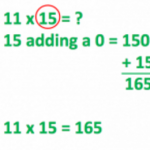Smartick is a fun way to learn math!I want to learn about: Mutiplication

Content about multiplication in elementary mathematics. Exercises, tutorials, problems, and teaching resources for elementary multiplication. Here you will find posts about multiplication to make learning mathematics easier and more fun.

Apr13

What is Multiplication: Steps to Learn and Understand Multiplication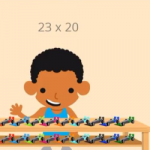What is multiplication? We will explain it in this post! Then we will show some examples using the steps that you should follow to complete multiplication problems, along with a video. What is Multiplication? Multiplication is a mathematical operation that is performed to calculate the result by adding the number as many times as indicated […]

Nov11

Multiplication of Decimals Using MoneyToday we are going to better understand the multiplication of decimals by using a very common manipulative material: money. It is going to help us understand why multiplying by 10 moves the decimal position to the right. Equivalence of Bills or Coins with Numbers First, we are going to establish the equivalence of each coin […]

Sep09

Alternative Methods for Multiplication: Russian and Hindu Methods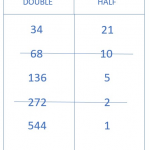In today’s post, we are going to learn two methods to solve multiplication problems that are different from the ones we are used to using. First, we are going to travel to Russia where we will learn how to multiply using the Russian Method. Then, we will continue on to India to learn the Hindu […]

Feb25

Pedagogical Justification of the Commutative Property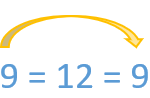In this post, we are going to justify, in a pedagogical sense, the conceptual base of the commutative property and it’s most basic and practical applications. The first thing we need to do is review the concept of the commutative property. For this I have linked some older blog posts about this topic: Applying the […]

Jan28

How to Perform Multiplication Problems with an Area Model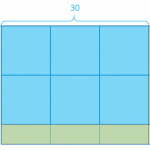In today’s post, we are going to learn how to solve multiplication problems using an area model. You will see how simple it is to understand this multiplication algorithm. Earlier with Smartick, we saw that we can represent multiplication with a rectangle whose sides have multiplication factors. Today I will teach you the area model, based […]

Jul02

The Relation between Multiplication and Division

Multiplication and division are closely related, given that division is the inverse operation of multiplication. When we divide, we look to separate into equal groups, while multiplication involves joining equal groups. In today’s post, we’re going to learn to use multiplication as a strategy to solve division problems, which will be really useful for daily life! We’ll start with a […]

Feb26

Learn Your Times Tables at a Glance with a 100 SquareIn today’s post we’re going to represent the results of the times tables in a “100 square”. Learning your times tables will be much easier with this visual aid! A “100 square” is nothing more than a table of the numbers 1 to 100 arranged in rows of 10 by 10, like this: We’re going to see what patterns we can create […]

Jan22

Different Methods of Multiplication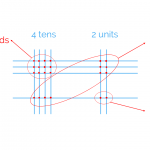In today’s post we’re going to find out how ancient cultures would have solved the multiplication we’ve chosen. It will help you to better understand how the classic multiplication algorithm works. I’m sure you’re already an expert in multiplication, but you might not understand exactly why it works the way it does. We’re going to look at Chinese and Egyptian methods […]

Dec29

Multiplication Tables: How to Work Them out Using a Grid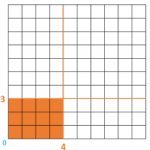We’ve already spent a lot of time talking about multiplication tables on the Smartick Blog. In previous posts, we’ve seen different ways of learning them. Take a look at the links below if you want to see any of them: Times tables to download and print: Downloadable tables that you can save and print. Now […]

Dec04

Some Tricks to Make Times Tables Shorter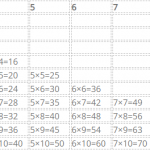In today’s post we’re going to review our times tables, while at the same time learning a trick to make them shorter. Before we start, you can review the times tables if you need to by clicking this link. Are you ready? Let’s start! Have you noticed that the numbers repeat across all the times tables? For example, […]

Nov10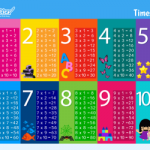Who said that times tables have to be boring black and white squares? In today’s post, we wanted to share some times tables that you can download and print to stick on your wall, keep in a folder, or have them on hand whenever and wherever you want. Learning your times tables is easy and fun. To […]